# Statistical Modelling and Machine Learning in R: Part 1 Lecture Notes

## Overview

In last session we learned about data wrangling and visualization. In this one, we’ll learn about statistical modelling and machine learning in R. Specifically, we’ll look at some supervised learning tasks (both regression and classification) and touch on some unsupervised learning at the end. We’ll discuss out-of-sample testing, regularization, and model selection via cross-validation. We’ll continue to add to your data wrangling toolkit by tackling some textual fields and looking at a natural language processing model.

## Some Modelling Concepts

Before diving into the code, let’s take a couple minutes to get oriented.

### Machine Learning and Modelling

We will be working with statistical and machine learning models. At its heart, machine learning is unknown function approximation - there is a relationship between the different columns of the data points which we do not know but would like to find out about. For most of the class, we’ll be looking at supervised learning models, in which the unknown function of interest is a mapping of predictor variables to response variables. We’ll conceptualize such models as having two parts:

1. The first part of modelling will be to specify a structure within which we’ll explore the relationship of predictors to response (e.g. we specify that the response variable depends linearly on the predictor).

2. Then we’ll use optimization over a data set to find the “best” model within the structural class we’ve specificed, where “best” means that the model minimizes some measure of the error between predicted and actual values.

We’ll also look briefly at unsupervised learning, where we don’t separate the data into predictors and response variables, but instead study the relationship of data points to each other (for example via clustering).

The focus of this course will be to get you familiar with the way R represents models and to provide examples of training and testing models. We hope that with this understanding in place, you’ll be able to generalize your skills from the models we’ll cover here to the vast world of models that’s available.

### Model Evaluation

It is important to ask, “How good is my model?” The answer is far from clear a lot of the time, since there are often multiple good explanations for the data and since structural assumptions on relationships between data points can be difficult to verify. However, simply speaking there are at least two uses for a model that result in two different ideas about what qualifies a model as “good.” First, you may care about explaining the relationship between variables (inference), in which case getting the right model structure is very important. However, you may care instead about predicting outcomes of one variable based on another, in which case verifying the exact mathematical relationship is less important. What becomes important in this second setting is validating your model on data other than the data you used to build the model. We’ll focus mainly on predictive quality, though we’ll look at a couple of ways to check explanatory power in the context of linear regression.

## Regression

Within the world of supervised learning, we can divide tasks into two parts. In settings where the response variable is continuous we call the modelling regression, and when the response is categorical we call it classification. We will begin with regression to understand what factors influence price in the AirBnB data set.

Let’s start by loading the data (after first setting the correct working directory). We’ll use the ‘listings.csv’ file for now. Since we’ll be wrangling and visualizing, we’ll also load the tidyverse package. (Instead of tidyverse, it also works to load tidyr, dplyr, and ggplot2 as we saw last session.)

listings = read.csv("listings.csv",stringsAsFactors = FALSE)
library(tidyverse)

### Linear Regression

As a review, we need to change the price column to a numeric form.

listings = listings %>% mutate(price = as.numeric(gsub("\\$|,","",price))) summary(listings$price) # Check to make sure things worked
##    Min. 1st Qu.  Median    Mean 3rd Qu.    Max.
##    10.0    85.0   150.0   173.9   220.0  4000.0

Now, which variables may be predictive of price? We can use names(listings) to get a look at all the variable names.

#### Data Preparation

Let’s begin by looking at the relationship between listings$accommodates and listings$price. As a first look:

plot(listings$accommodates,listings$price) # Note: I use base R instead of ggplot2 for the really simple first-blush plots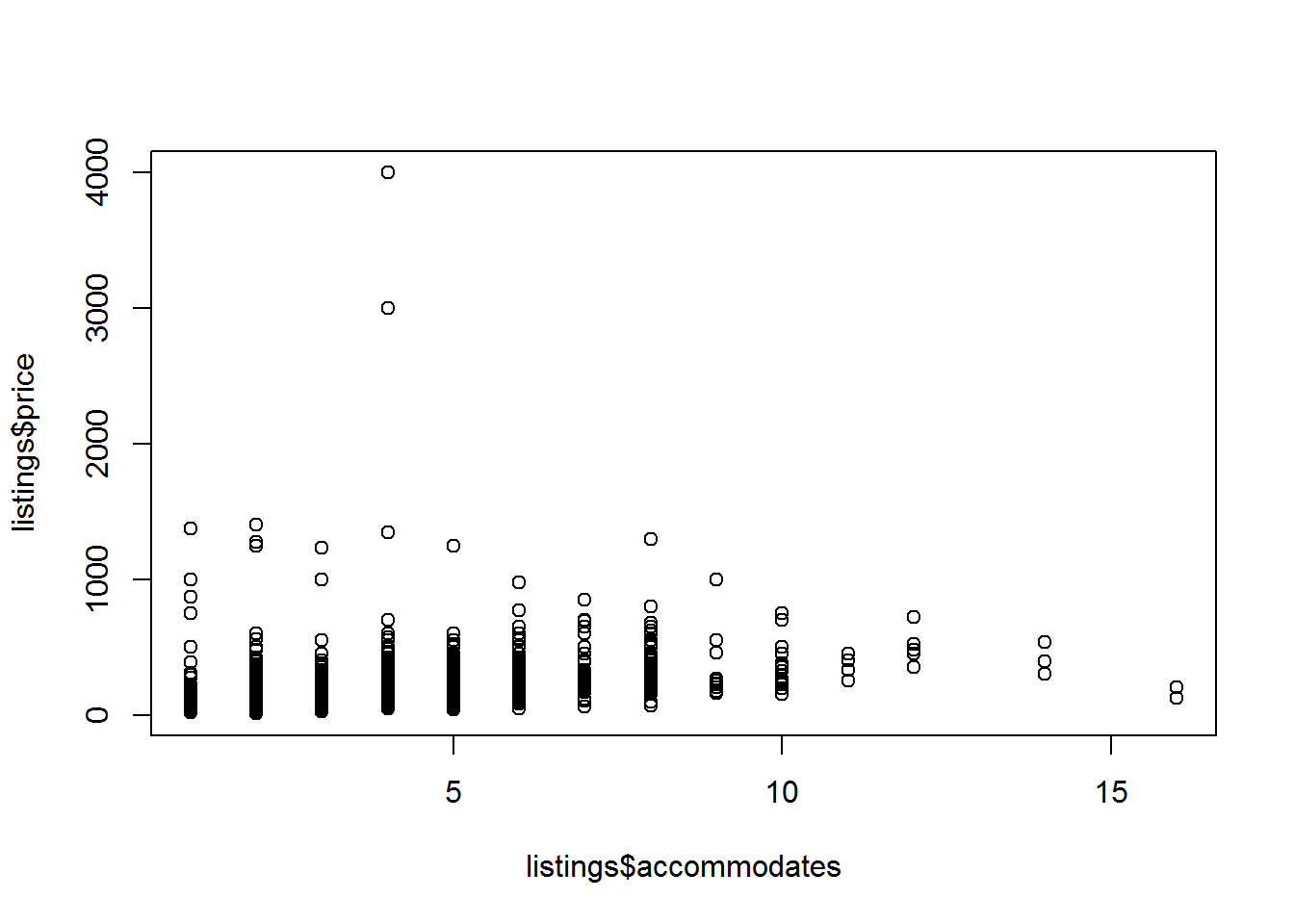Looks like there are some outliers on both axes. There are fancier ways to deal with this statistically, but for today let’s just get rid of the outliers and fit a model on the cleaner data:

listings_for_lm = listings %>%
filter(accommodates <= 10, price <=1000)

Let’s take another look:

plot(listings_for_lm$accommodates,listings_for_lm$price)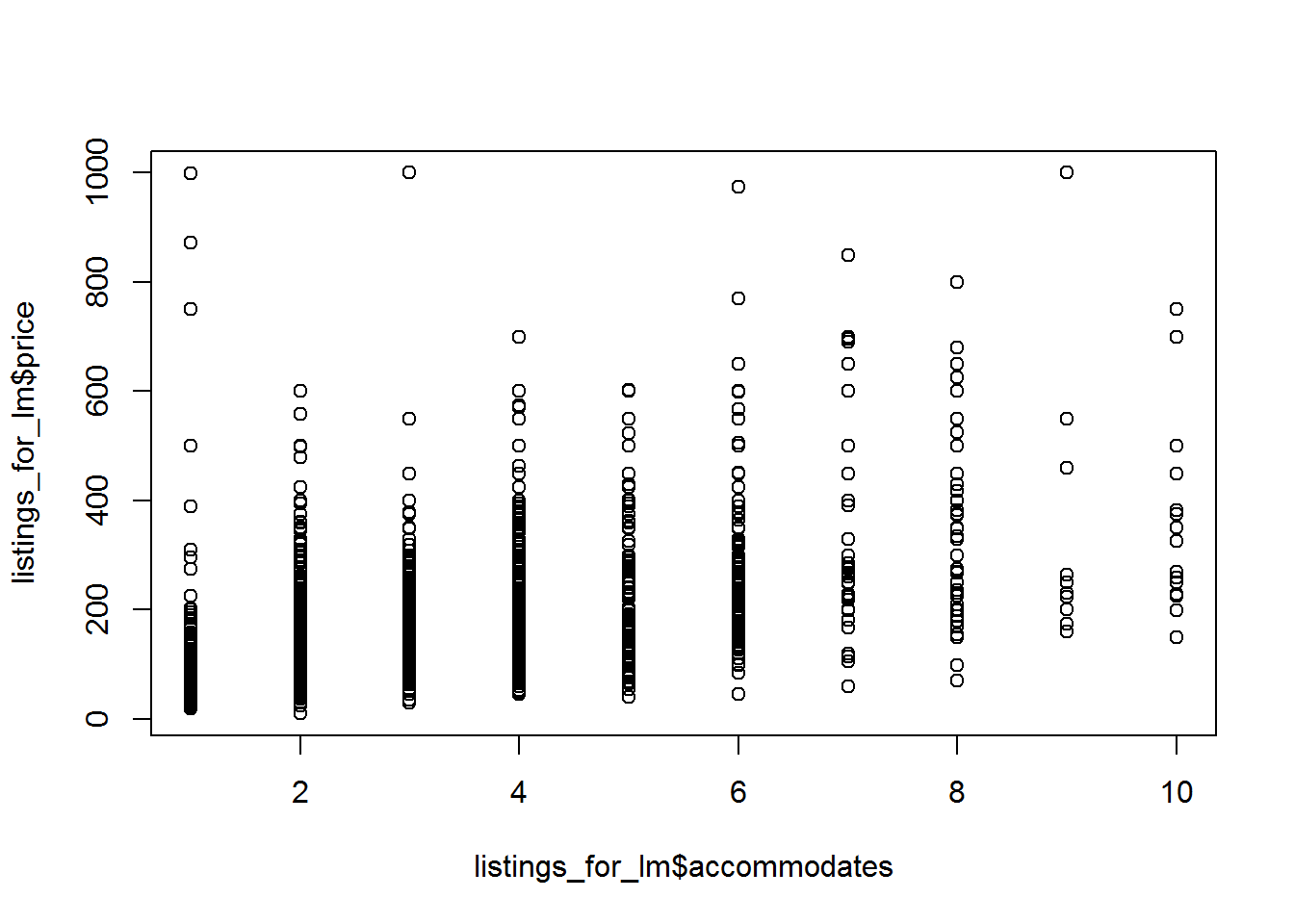You may argue that it’s still a bit messy, but let’s see what we can do with a linear model. Since we care about prediction accuracy, we’ll reserve a portion of our data to be a test set. There are lots of ways to do this. We’ll use the modelr package, which is part of the tidyverse.

library(modelr) # Comes with tidyverse installation, but doesn't automatically load with the library(tidyverse) call
set.seed(445)
listings_for_lm = listings_for_lm %>%
resample_partition(c(train=0.7,test=0.3))

The object listings_for_lm is now a list with two elements: train and test.

#### Model Fitting

In R, we specify a model structure and then use the corresponding function to tell R to optimize for the best-fitting model. For linear regression, the function is lm():

lm_price_by_acc = lm(price ~ accommodates,data=listings_for_lm$train) # We'll talk more about the '~' notation soon Let’s check out the lm_price_by_acc object: names(lm_price_by_acc) ##  "coefficients" "residuals" "effects" "rank" ##  "fitted.values" "assign" "qr" "df.residual" ##  "xlevels" "call" "terms" "model" The object lm_price_by_acc is a list of a bunch of relevant information generated by the lm() function call. We can use the $ to view different elements, for example

lm_price_by_acc$call ## lm(formula = price ~ accommodates, data = listings_for_lm$train)

So, it stores the function call in one of the list elements. But this isn’t the most useful way to check out the model fit. The function summary() is overloaded for many different objects and often gives a useful snapshot of the model:

summary(lm_price_by_acc)
##
## Call:
## lm(formula = price ~ accommodates, data = listings_for_lm$train) ## ## Residuals: ## Min 1Q Median 3Q Max ## -301.85 -53.76 -15.03 39.47 684.70 ## ## Coefficients: ## Estimate Std. Error t value Pr(>|t|) ## (Intercept) 47.983 3.720 12.90 <2e-16 *** ## accommodates 40.386 1.089 37.07 <2e-16 *** ## --- ## Signif. codes: 0 '***' 0.001 '**' 0.01 '*' 0.05 '.' 0.1 ' ' 1 ## ## Residual standard error: 89.57 on 2490 degrees of freedom ## Multiple R-squared: 0.3556, Adjusted R-squared: 0.3554 ## F-statistic: 1374 on 1 and 2490 DF, p-value: < 2.2e-16 There we go, this is more useful! First, let’s look at the section under “Coefficients”. Notice that R automatically adds an intercept term unless you tell it not to (we’ll see how to do this later). In the “estimate” column, we see that the point estimates for the linear model here say that the price is$55.20 plus $37.79 for every person accommodated. Notice the ’***’ symbols at the end of the “(Intercept)” and “accommodates” rows. These indicate that according to a statistical t-test, both coefficients are extremely significantly different than zero, so things are okay from an inference perspective. #### Model Evaluation As another check on inference quality, let’s plot the fitted line. There are some nifty functions in the modelr package that make interacting with models easy in the tidyr and dplyr setting. We’ll use modelr::add_predictions() here. as.data.frame(listings_for_lm$train) %>%
ggplot(aes(x=accommodates)) +
geom_point(aes(y=price)) +
geom_line(aes(y=pred), color='red')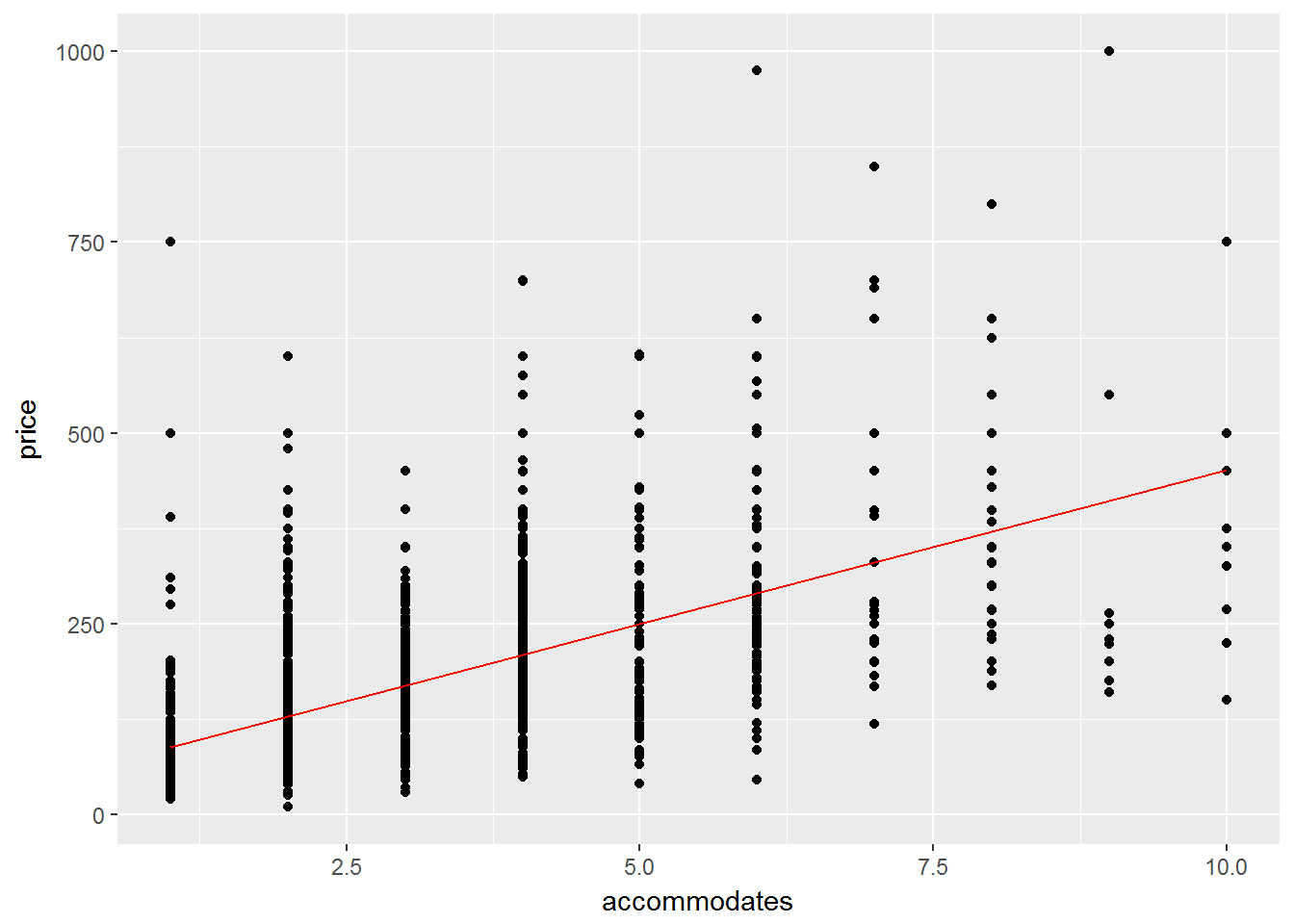Nice. We can also remove the linear trend and check the residual uncertainty, which we’ll do here using modelr::add_residuals(). This is helpful to make sure that the residual uncertainty looks like random noise rather than systematic bias.

as.data.frame(listings_for_lm$train) %>% add_residuals(lm_price_by_acc,var="resid") %>% ggplot(aes(x=accommodates,y=resid)) + geom_point()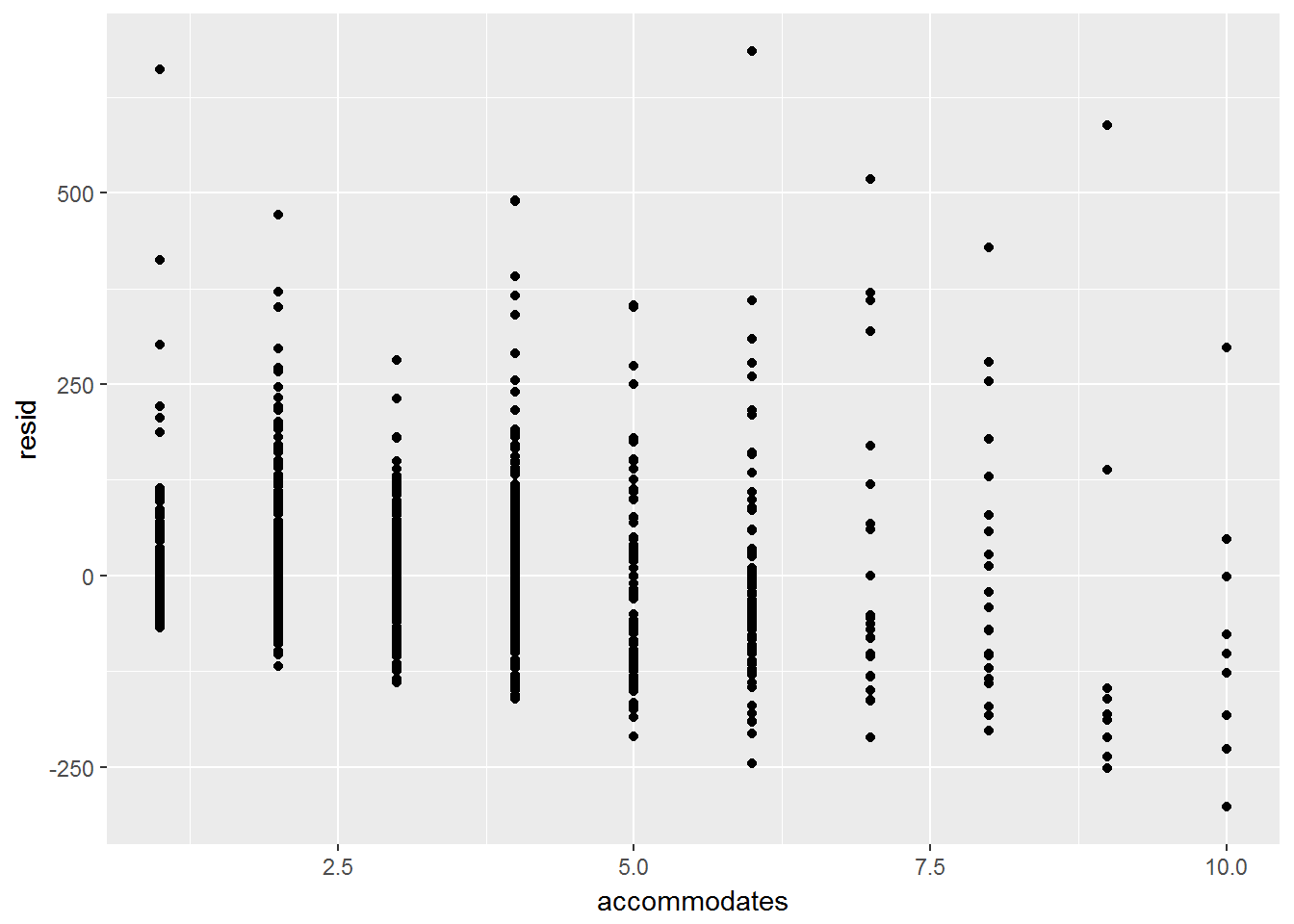Since we have finitely many values, maybe box plots tell a better story: as.data.frame(listings_for_lm$train) %>%
group_by(as.factor(accommodates)) %>%
ggplot(aes(x=as.factor(accommodates),y=resid)) + geom_boxplot()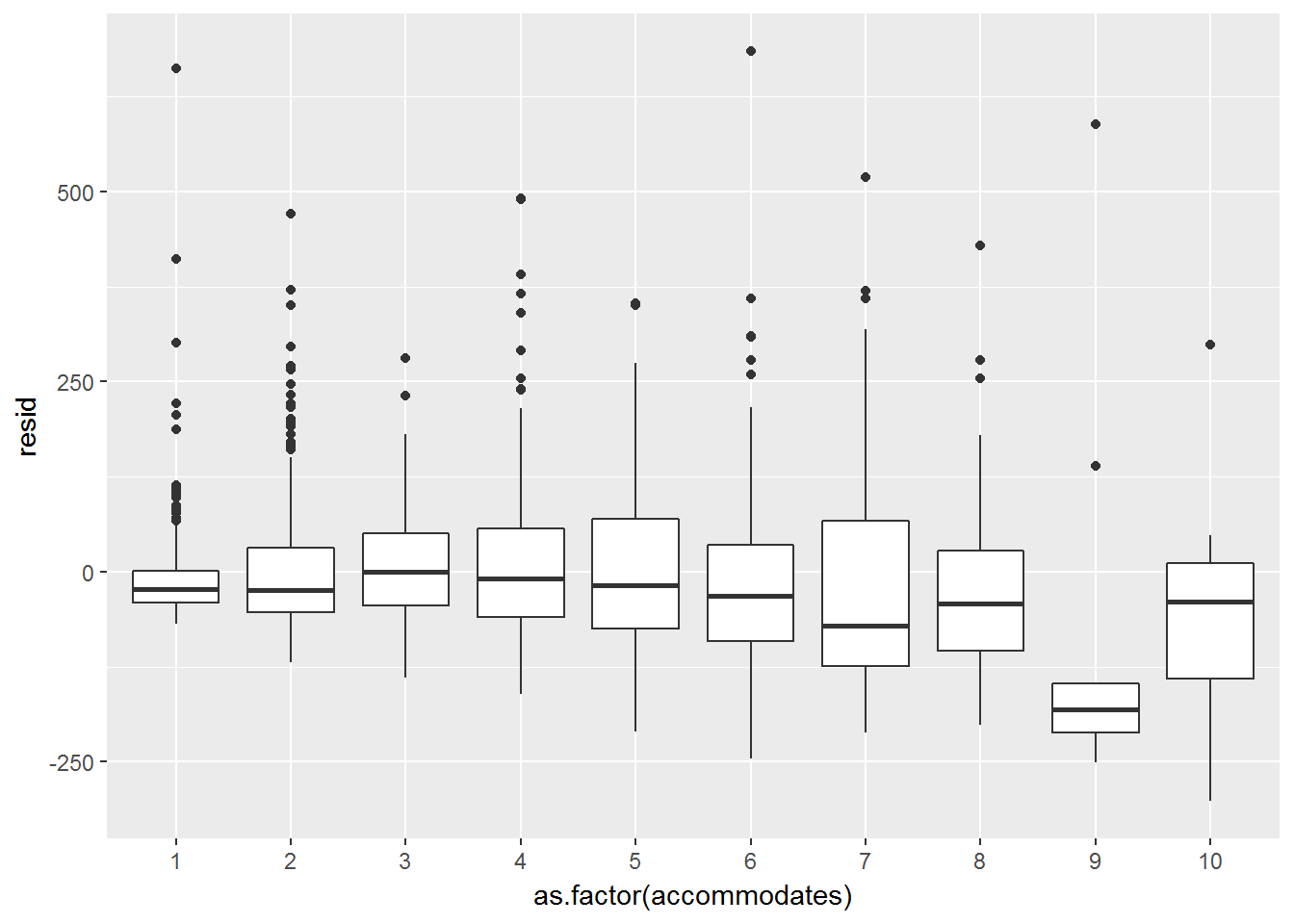Things are pretty centered around zero, with the exception of 9- & 10-person accommodations. Maybe the model doesn’t apply so well here and we could refine it in a later modelling iteration.

Let’s now take a look at out-of-sample performance. We’ll plot the predictions versus the actuals as we did before, but this time for the test data.

as.data.frame(listings_for_lm$test) %>% add_predictions(lm_price_by_acc) %>% ggplot(aes(x=accommodates)) + geom_point(aes(y=price)) + geom_line(aes(y=pred), color='red')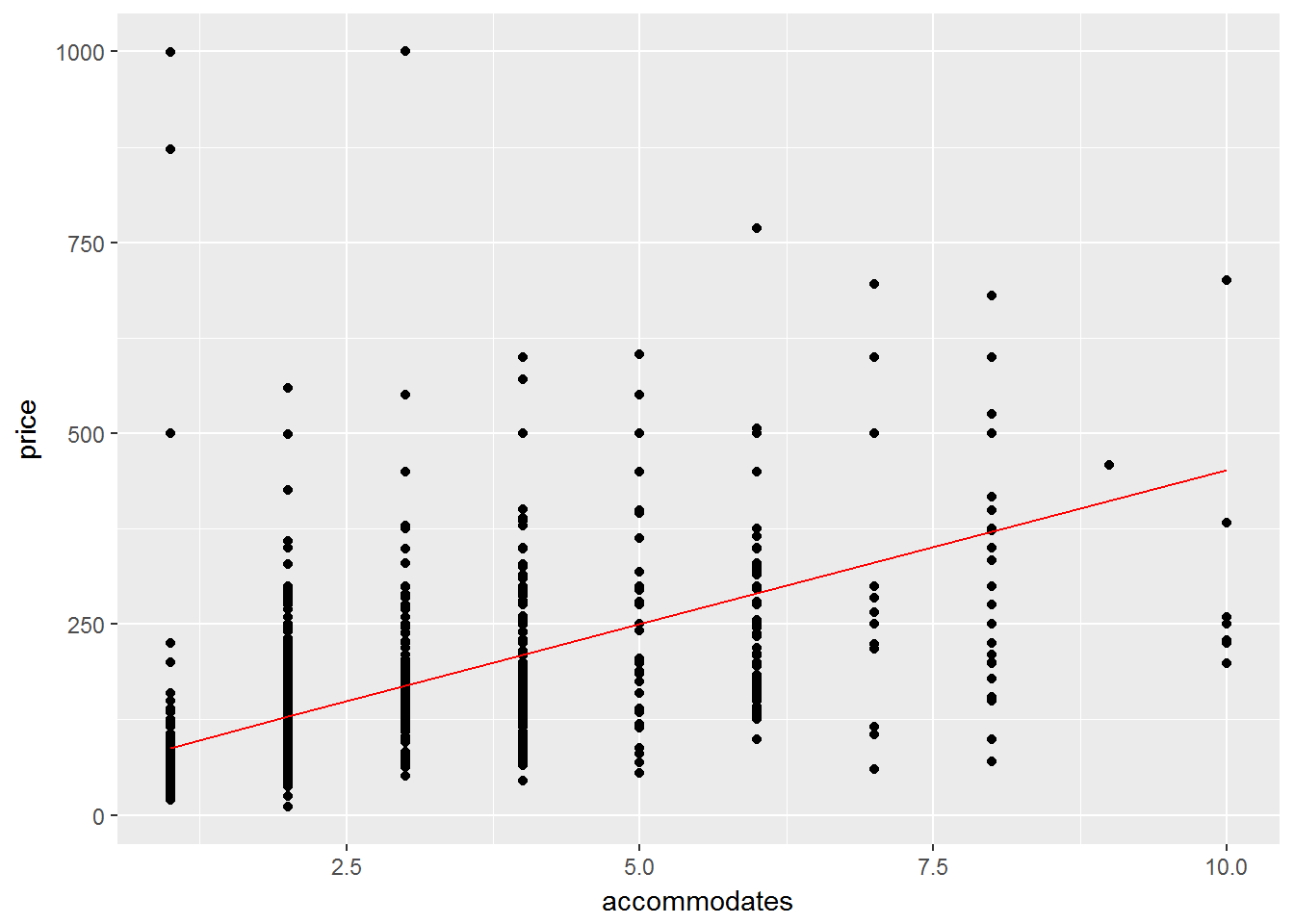Now, what if we wanted to quantify how well the model predicts these out-of-sample values? There are several metrics to aggregate the prediction error. We’ll look at two: • Root Mean-squared Error (RMSE): $$\sqrt{\sum_{t=1}^n (\hat{y}_t - y_t)^2/n}$$ • Mean Absolute Error (MAE): $$\sum_{t=1}^n |\hat{y}_t - y_t|/n$$ In both, $$\hat{y}_t$$ is the predicted value for test observation $$t$$, $$y_t$$ is the actual value, and $$n$$ is the size of the test set. You could calculate these pretty easily with a line or two of code, and we’ll do so later on, but modelr has built-in functions to do this for you automatically: rmse(lm_price_by_acc,listings_for_lm$test)
##  100.5159
mae(lm_price_by_acc,listings_for_lm$test) ##  68.62211 The units on both measurements are$, and since they’re measures of error, lower is better. These values don’t help much on their own, but they come in handy when comparing different models, which we’ll do next after a quick review.

#### Summary and More about Formulas

First, let’s review the pattern, because it can be generalized to a whole bunch of more complex models. We asked the questions: How does listing price depend on the number of people it accommodates? How well does accommodation size predict price? Since we were interested in prediction, we reserved part of our data as a test set. We then chose to use a linear model to answer these questions, and found the corresponding function lm(). This function, and modelling functions in general, takes as arguments

• Data on the response and predictor variables, usually through a formula object
• Model parameters (in the case of lm(), we used all the default values)

R then automatically found the “best” linear model by computing the least squares estimate, and returned a lm object, which was a list including information about

• Fitted coefficients
• Residuals
• Statistical significance
• And more…

We interacted with the model to evaluate goodness-of-fit and out-of-sample performance. In our case, we used the modelr and dplyr framework to do this cleanly.

We didn’t say too much about the price ~ accommodates syntax. Many modelling functions in R take formulas as arguments, together with a data argument. The data argument specifies the data frame, and the formula argument tells the model which are the responses and which are the predictors. We’ll play around with formulas in the exercises, but here are a few helpful pointers:

• The ~ separates the response (on the left) from the predictors (on the right)
• Predictors are separated with a +
• Use . on the right-hand side to include all predictors in a given data frame
• You can also use .-x to include all predictors except x
• To include interactions between variables, use the * symbol. For example: y ~ x + z + x*z expresses the form: “regress y on x, z, and x interacted with z
• To exclude the intercept term, include -1 or +0 on the right-hand side

For more detailed info, see https://stat.ethz.ch/R-manual/R-devel/library/stats/html/formula.html.

### Other Models - Splines

Now that we’ve identified the pattern, let’s look at another type of model: splines. Without going into all the mathematical details, splines are (roughly) one way of fitting a higher-degree polynomial to the data. Let’s look at what happens when we allow a quadratic and cubic fit in the price by accomodation size setting.

library(splines)
lm2 = lm(price ~ ns(accommodates,2),data=listings_for_lm$train) lm3 = lm(price ~ ns(accommodates,3),data=listings_for_lm$train)

We can again look inside these model objects using summary():

summary(lm2)
##
## Call:
## lm(formula = price ~ ns(accommodates, 2), data = listings_for_lm$train) ## ## Residuals: ## Min 1Q Median 3Q Max ## -249.53 -53.55 -16.84 33.26 687.19 ## ## Coefficients: ## Estimate Std. Error t value Pr(>|t|) ## (Intercept) 76.840 3.868 19.86 <2e-16 *** ## ns(accommodates, 2)1 357.779 10.366 34.52 <2e-16 *** ## ns(accommodates, 2)2 267.484 15.112 17.70 <2e-16 *** ## --- ## Signif. codes: 0 '***' 0.001 '**' 0.01 '*' 0.05 '.' 0.1 ' ' 1 ## ## Residual standard error: 89.25 on 2489 degrees of freedom ## Multiple R-squared: 0.3604, Adjusted R-squared: 0.3599 ## F-statistic: 701.3 on 2 and 2489 DF, p-value: < 2.2e-16 We can also plot the out-of-sample performance of each separately: as.data.frame(listings_for_lm$test) %>%
ggplot(aes(x=accommodates)) +
geom_point(aes(y=price)) +
geom_line(aes(y=pred), color='red')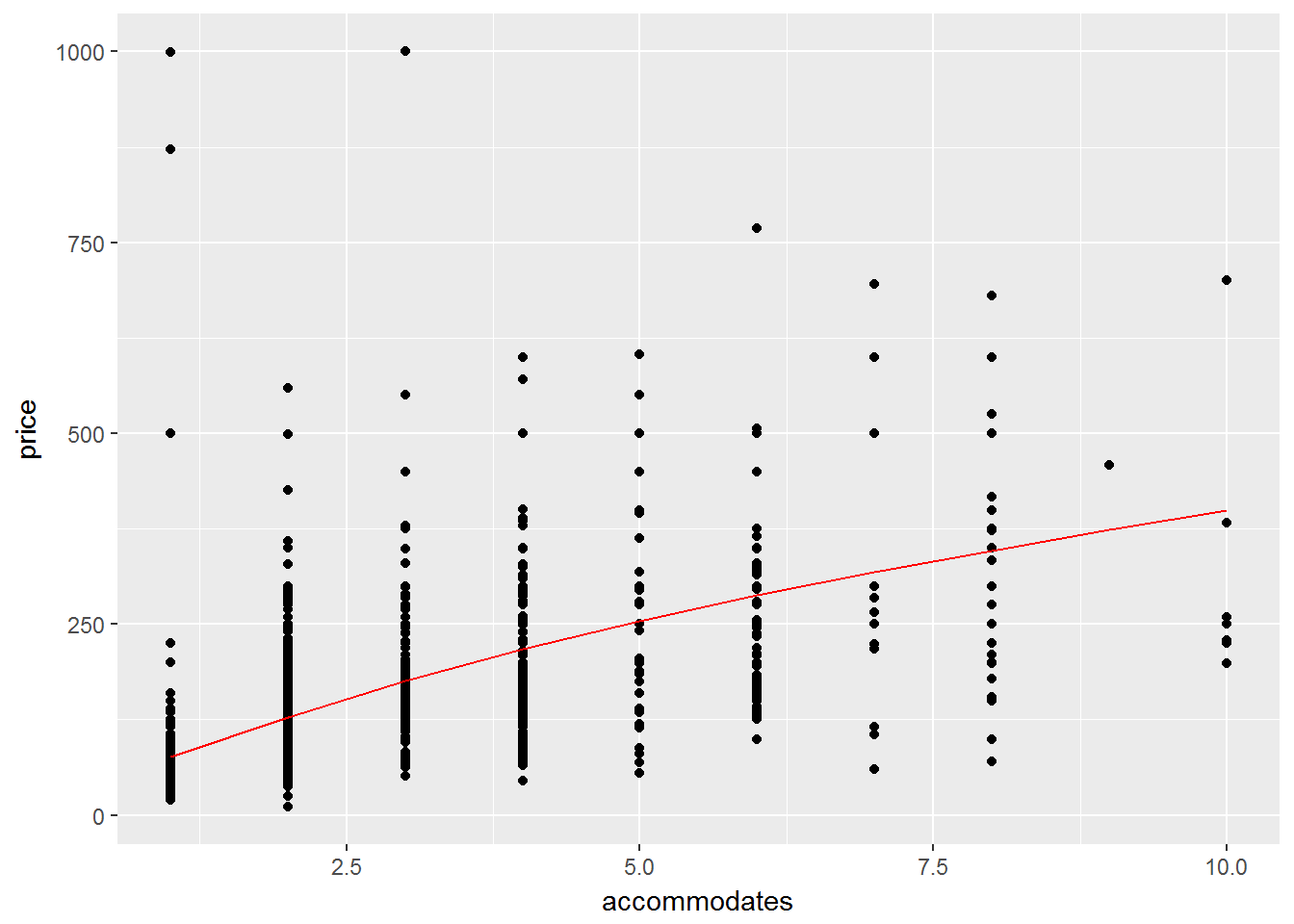To plot the linear, quadratic, and cubic models all at once, we’ll use gather_predictions(). This function makes a big long column of predictions, with another column to tell which model generated which prediction (so that the data is in long or tidy format):

as.data.frame(listings_for_lm$test) %>% gather_predictions(lm_price_by_acc,lm2,lm3) %>% ggplot(aes(x=accommodates)) + geom_point(aes(y=price)) + geom_line(aes(y=pred,group=model,color=model))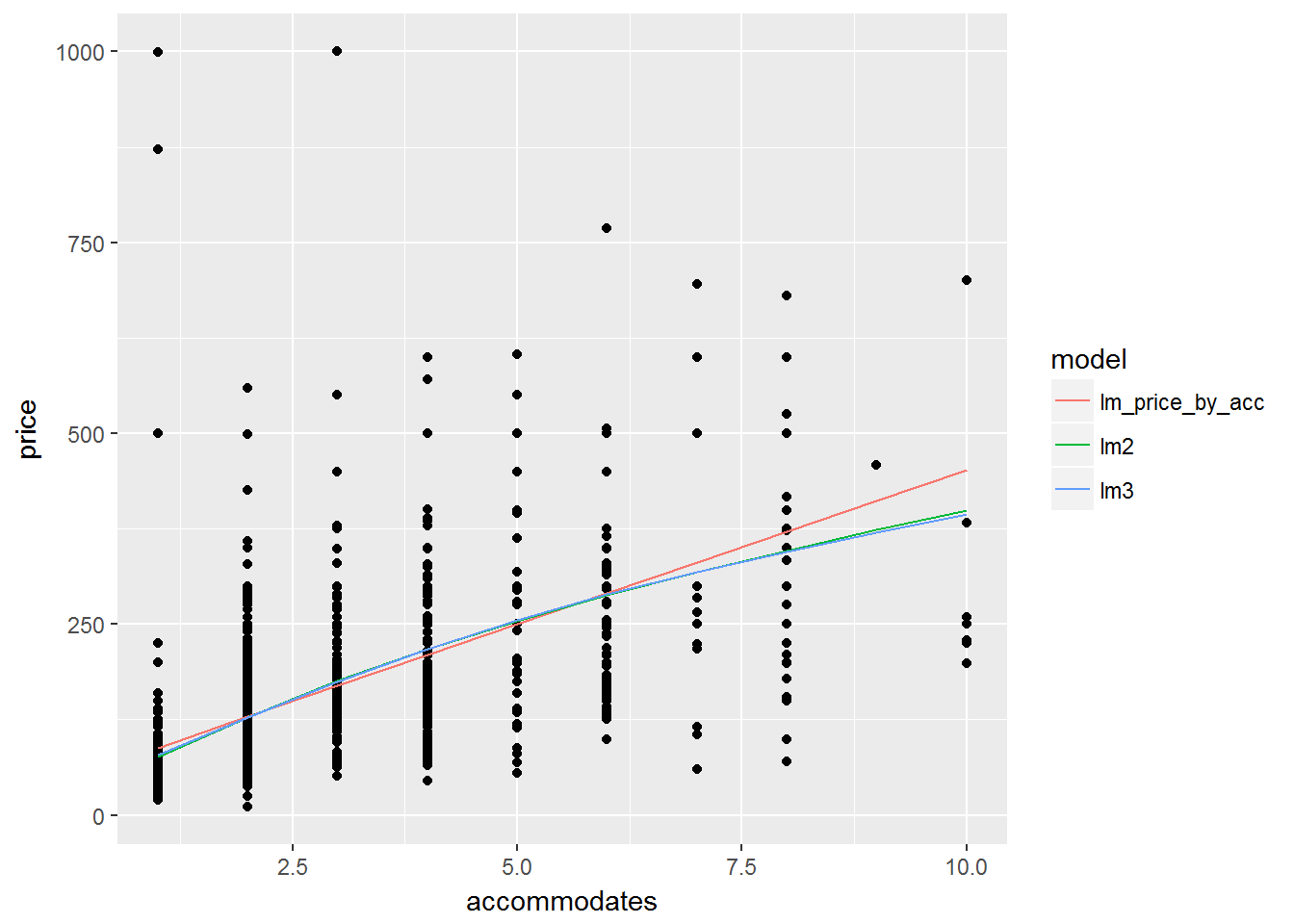In this case, all the models look reasonable. The quadratic and cubic models are hard to distinguish from each other. Let’s compare the RMSE and MAE: rmse(lm_price_by_acc,listings_for_lm$test)
##  100.5159
rmse(lm2,listings_for_lm$test) ##  99.88911 rmse(lm3,listings_for_lm$test)
##  99.8826
mae(lm_price_by_acc,listings_for_lm$test) ##  68.62211 mae(lm2,listings_for_lm$test)
##  68.10766
mae(lm3,listings_for_lm$test) ##  68.14386 All values are roughly the same. The higher-order models give a bit of advantage, but not much. The fact that we only gain slightly from a more complex model isn’t surprising, given that we only have data for 10 distinct values of the accommodates variable. In fact, in some cases more complex models perform worse since they overfit to the data in the training set. ## Model Selection and Tuning: Penalized Regression Let’s work a bit harder on predicting price, this time using more than one predictor. In fact, we’ll add a bunch of predictors to the model and see what happens. As one set of predictors, the column listings$amenities looks interesting:

listings %>% select(amenities) %>% head()
##                                                                                                                                                                                                                                                                                                                      amenities
## 1                                                                                               {TV,"Wireless Internet",Kitchen,"Free Parking on Premises","Pets live on this property",Dog(s),Heating,"Family/Kid Friendly",Washer,Dryer,"Smoke Detector","Fire Extinguisher",Essentials,Shampoo,"Laptop Friendly Workspace"}
## 2                               {TV,Internet,"Wireless Internet","Air Conditioning",Kitchen,"Pets Allowed","Pets live on this property",Dog(s),Heating,"Family/Kid Friendly",Washer,Dryer,"Smoke Detector","Carbon Monoxide Detector","Fire Extinguisher",Essentials,Shampoo,"Lock on Bedroom Door",Hangers,"Hair Dryer",Iron}
## 3 {TV,"Cable TV","Wireless Internet","Air Conditioning",Kitchen,"Free Parking on Premises",Heating,Washer,Dryer,"Smoke Detector","Carbon Monoxide Detector","First Aid Kit","Safety Card",Essentials,Shampoo,"Lock on Bedroom Door","translation missing: en.hosting_amenity_49","translation missing: en.hosting_amenity_50"}
## 4         {TV,Internet,"Wireless Internet","Air Conditioning",Kitchen,"Free Parking on Premises",Gym,Breakfast,"Indoor Fireplace",Heating,Washer,Dryer,"Smoke Detector","Carbon Monoxide Detector","First Aid Kit","Safety Card","Fire Extinguisher",Essentials,Shampoo,Hangers,"Hair Dryer",Iron,"Laptop Friendly Workspace"}
## 5                                                                                                                                         {Internet,"Wireless Internet","Air Conditioning",Kitchen,Breakfast,Heating,"Smoke Detector","Carbon Monoxide Detector","First Aid Kit",Essentials,Shampoo,Hangers,"Hair Dryer",Iron}
## 6                                                                                                            {"Cable TV","Wireless Internet","Air Conditioning",Kitchen,"Free Parking on Premises","Pets live on this property",Cat(s),Heating,"Family/Kid Friendly","Smoke Detector","Carbon Monoxide Detector",Hangers,Iron}

This could be good predictive information if we can separate out which listing has which amenity. Our goal here is to turn the amenities column into many columns, one for each amenity, and with logical values indicating whether each listing has each amenity. This is just a bit tricky, so I’ve written a function called clean_amenities that will do this for us. We need to source() the file that has this function in it, and then we’ll call it on the listings data frame.

source("../3_modeling_and_ml/clean_amenities.R")
listings = clean_amenities(listings)

In total, we’ll use all of these predictors:

• accommodates
• property_type
• review_scores_rating
• neighbourhood_cleansed
• accommodates*room_type
• property_type*neighbourhood_cleansed
• review_scores_rating*neighbourhood_cleansed
• accommodates*review_scores_rating
• All columns created from the amenities column

Note that whenever we include a non-numeric (or categorical) variable, R is going to create one indicator variable for all but one unique value of the variable. We’ll see this in the output of lm().

First, let’s clean up the data by getting rid of missing values and outliers. For categorical variables, we will remove all categories with only a few observations. Finally, we’ll separate again into training and test sets.

listings_big = listings %>%
filter(!is.na(review_scores_rating),
accommodates <= 10,
property_type %in% c("Apartment","House","Bed & Breakfast","Condominium","Loft","Townhouse"),
!(neighbourhood_cleansed %in% c("Leather District","Longwood Medical Area")),
price <= 1000) %>%
select(price,accommodates,room_type,property_type,review_scores_rating,neighbourhood_cleansed,starts_with("amenity"))

set.seed(144)
listings_big_lm = listings_big %>%
resample_partition(c(train=0.7,test=0.3))

To get R to learn the model, we need to pass it a formula. We don’t want to write down all those amenity variables by hand. Luckily, we can use the paste() function to string all the variable names together, and then the as.formula() function to translate a string into a formula.

all_amenities = as.data.frame(listings_big_lm$train) %>% select(starts_with("amenity")) %>% names() amenities_string = paste(all_amenities,collapse="+") amenities_string # Taking a look to make sure things worked ##  "amenity_TV+amenity_Wireless_Internet+amenity_Kitchen+amenity_Free_Parking_on_Premises+amenity_Pets_live_on_this_property+amenity_Dogs+amenity_Heating+amenity_Family_Kid_Friendly+amenity_Washer+amenity_Dryer+amenity_Smoke_Detector+amenity_Fire_Extinguisher+amenity_Essentials+amenity_Shampoo+amenity_Laptop_Friendly_Workspace+amenity_Internet+amenity_Air_Conditioning+amenity_Pets_Allowed+amenity_Carbon_Monoxide_Detector+amenity_Lock_on_Bedroom_Door+amenity_Hangers+amenity_Hair_Dryer+amenity_Iron+amenity_Gym+amenity_Breakfast+amenity_Indoor_Fireplace+amenity_First_Aid_Kit+amenity_Safety_Card+amenity_Cable_TV+amenity_Cats+amenity_x24Hour_Checkin+amenity_Hot_Tub+amenity_Other_pets+amenity_Buzzer_Wireless_Intercom+amenity_Washer___Dryer+amenity_Smoking_Allowed+amenity_Suitable_for_Events+amenity_Wheelchair_Accessible+amenity_Elevator_in_Building+amenity_Doorman+amenity_Pool+amenity_Paid_Parking_Off_Premises+amenity_Free_Parking_on_Street" big_formula = as.formula(paste("price ~ accommodates + accommodates*room_type + property_type + neighbourhood_cleansed + property_type*neighbourhood_cleansed + review_scores_rating*neighbourhood_cleansed + accommodates*review_scores_rating",amenities_string,sep="+")) Now we can use the lm() function: big_price_lm = lm(big_formula,data=listings_big_lm$train)

We won’t look at the summary because there are so many predictors. What happens when we compare in-sample and out-of-sample prediction performance?

rmse(big_price_lm,listings_big_lm$train) # In-sample ## Warning in predict.lm(model, data): prediction from a rank-deficient fit ## may be misleading ##  63.92064 rmse(big_price_lm,listings_big_lm$test) # Out-of-sample
## Warning in predict.lm(model, data): prediction from a rank-deficient fit
## may be misleading
##  76.71424

We’ve got an overfitting problem here, meaning that the training error is smaller than the test error. The model is too powerful for the amount of data we have. Note that R recognizes this by giving warnings about a “rank-deficient fit.”

### Regularized/Penalized Regression

But is there still a way to use the info from all these variables without overfitting? Yes! One way to do this is by regularized, or penalized, regression.

Mathematically, we add a term to the optimization problem that we’re solving when fitting a model, a term which penalizes models that get too fancy without enough data. If we call $$\beta$$ the coefficient vector that we’d like to learn about for linear regression, then the regular regression we’ve worked with looks like $\min_\beta \sum_{t=1}^n (y_t-x_t^T\beta)^2,$ but penalized regression looks like $\min_\beta \sum_{t=1}^n (y_t-x_t^T\beta)^2 + \lambda ||\beta||.$

There are two types of flexibility within this framework that I’ll mention:

• Choice of norm, a structural decision, and
• Choice of $$\lambda$$, a parametric decision.

Two natural choices of norm are the Euclidean 1- and 2-norms. When we use the 2-norm, it’s often called “ridge regression.” We’ll focus today on the 1-norm, or “LASSO regression”. On a very simple level, both types of regression shrink all the elements of the unconstrained $$\beta$$ vector towards zero, some more than others in a special way. LASSO shrinks the coefficients so that some are equal to zero. This feature is nice because it helps us interpret the model by getting rid of the effects of many of the variables.

To do LASSO, we’ll use the glmnet package. Of note, this package doesn’t work very elegantly with the tidyverse since it uses matrix representations of the data rather than data frame representations. However, it does what it does quite well, and will give us a chance to see some base R code. Let’s load the package and check out the function glmnet(). We can see the documentation from the command line using ?glmnet.

library(glmnet)

Notice that glmnet() doesn’t communicate with the data via formulas. Instead, it wants a matrix of predictor variables and a vector of values for the variable we’re trying to predict, including all the categorical variables that R automatically expanded into indicator variables. Fortunately, R has a model.matrix() function which takes a data frame and gets it into the right form for glmnet() and other functions with this type of input.

Notice also that there’s a way to specify lambda manually. Since we haven’t discussed choosing lambda yet, let’s just accept the default for now and see what we get.

x = model.matrix(~ .-price + accommodates*room_type + property_type*neighbourhood_cleansed + review_scores_rating*neighbourhood_cleansed + accommodates*review_scores_rating,data=as.data.frame(listings_big_lm$train)) y = as.vector(as.data.frame(listings_big_lm$train)$price) lasso_price = glmnet(x,y) This time the summary() function isn’t quite as useful: summary(lasso_price) ## Length Class Mode ## a0 100 -none- numeric ## beta 21000 dgCMatrix S4 ## df 100 -none- numeric ## dim 2 -none- numeric ## lambda 100 -none- numeric ## dev.ratio 100 -none- numeric ## nulldev 1 -none- numeric ## npasses 1 -none- numeric ## jerr 1 -none- numeric ## offset 1 -none- logical ## call 3 -none- call ## nobs 1 -none- numeric It does give us some info, though. Notice that “lambda” is a vector of length 88. The glmnet() function has defined 88 different values of lambda and found the corresponding optimal beta vector for each one! We have 88 different models here. Let’s look at some of the coefficients for the different models. We’ll start with one where lambda is really high: lasso_price$lambda
##  68.1282
nnzero(lasso_price$beta[,1]) # How many coefficients are nonzero? ##  0 Here the penalty on the size of the coefficients is so high that R sets them all to zero. Moving to some smaller lambdas: lasso_price$lambda
##  29.49107
lasso_price$beta[which(lasso_price$beta[,10] != 0),10]
##             room_typePrivate room accommodates:review_scores_rating
##                       -31.0357907                         0.1966795
lasso_price$lambda ##  11.63189 lasso_price$beta[which(lasso_price$beta[,20] != 0),20] ## room_typePrivate room ## -34.7310901 ## neighbourhood_cleansedBack Bay ## 10.2454032 ## amenity_TVTRUE ## 2.4733494 ## amenity_Free_Parking_on_PremisesTRUE ## -3.7078042 ## amenity_WasherTRUE ## 0.2888948 ## amenity_Air_ConditioningTRUE ## 3.4620595 ## amenity_Indoor_FireplaceTRUE ## 5.0209555 ## amenity_Cable_TVTRUE ## 9.2480922 ## amenity_Elevator_in_BuildingTRUE ## 11.1411059 ## accommodates:room_typePrivate room ## -6.2955671 ## property_typeCondominium:neighbourhood_cleansedFenway ## 5.0995782 ## accommodates:review_scores_rating ## 0.2773798 And, to see the whole path of lambdas: plot.glmnet(lasso_price,xvar="lambda")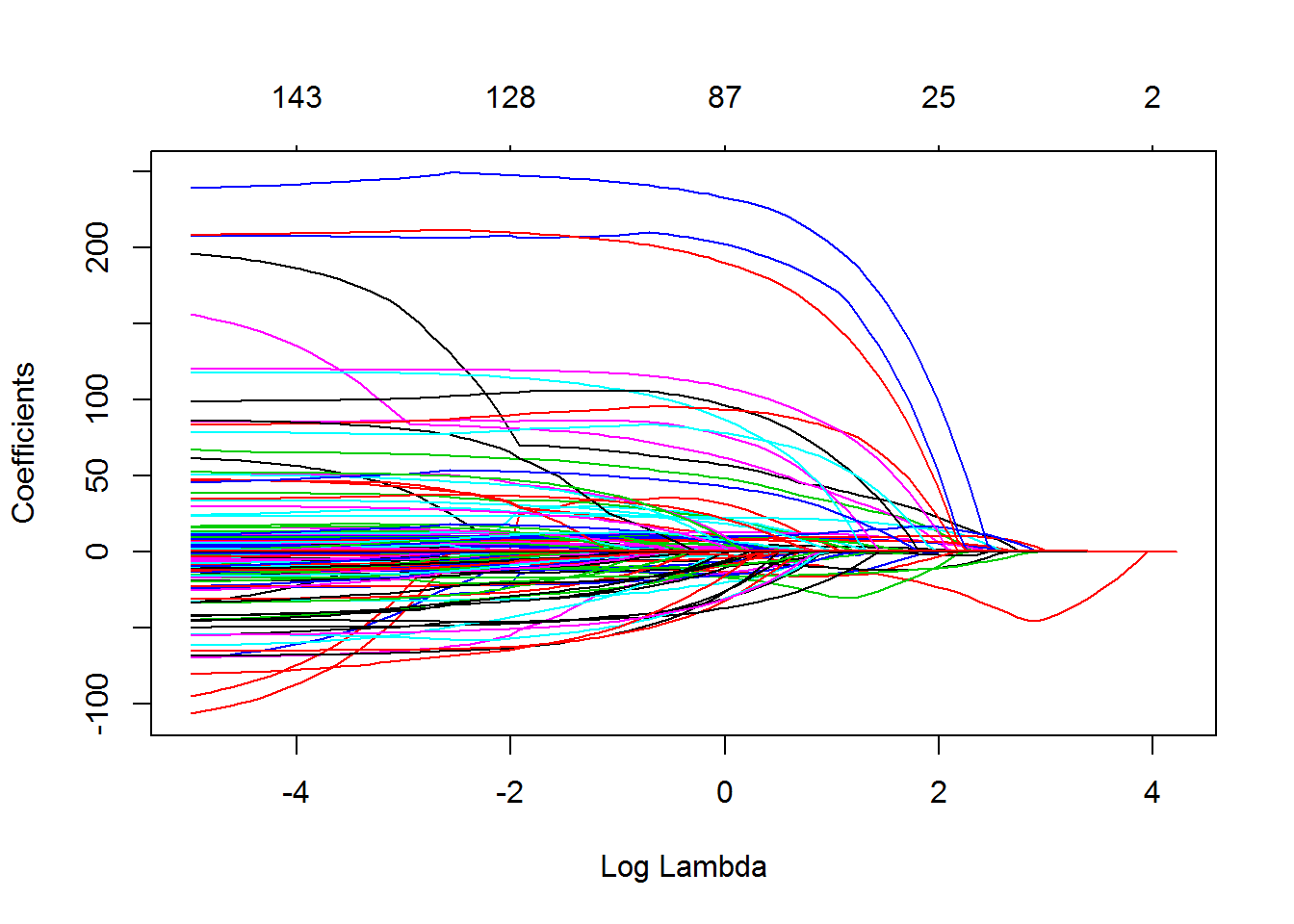Here, each line is one variable. The plot is quite messy with so many variables, but it gives us the idea. As lambda shrinks, the model adds more and more nonzero coefficients. ### Cross Validation How do we choose which of the 88 models to use? Or in other words, how do we “tune” the $$\lambda$$ parameter? We’ll use a similar idea to the training-test set split called cross-validation. The idea behind cross-validation is this: what if we trained our family of models (in this case 88) on only some of the training data and left out some other data points? Then we could use those other data points to figure out which of the lambdas works best out-of-sample. So we’d have a training set for training all the models, a validation set for choosing the best one, and a test set to evaluate performance once we’ve settled on a model. There’s just one other trick: since taking more samples reduces noise, could we somehow take more validation set samples? Here’s where cross-validation comes in. We divide the training data into groups called folds, and then for each fold repeat the train-validate procedure on the remaining training data and then use the current fold as a validation set. We then average the performance of each model on each fold and pick the best one. This is a very common resampling method that applies in lots and lots of settings. Lucky for us the glmnet package has a very handy function called cv.glmnet() which does the entire process automatically. Let’s look at the function arguments using ?cv.glmnet. The relevant arguments for us right now are • x, the matrix of predictors • y, the response variable • nfolds, the number of ways to split the training set (defaults to 10) • type.measure, the metric of prediction quality. It defaults to mean squared error, the square of RMSE, for linear regression Let’s do the cross-validation: lasso_price_cv = cv.glmnet(x,y) summary(lasso_price_cv) # What does the model object look like? ## Length Class Mode ## lambda 86 -none- numeric ## cvm 86 -none- numeric ## cvsd 86 -none- numeric ## cvup 86 -none- numeric ## cvlo 86 -none- numeric ## nzero 86 -none- numeric ## name 1 -none- character ## glmnet.fit 12 elnet list ## lambda.min 1 -none- numeric ## lambda.1se 1 -none- numeric Notice the “lambda.min”. This is the best lambda as determined by the cross validation. “lambda.1se” is the largest lambda such that the “error is within 1 standard error of the minimum.” There’s another automatic plotting function for cv.glmnet() which shows the error for each model: plot.cv.glmnet(lasso_price_cv)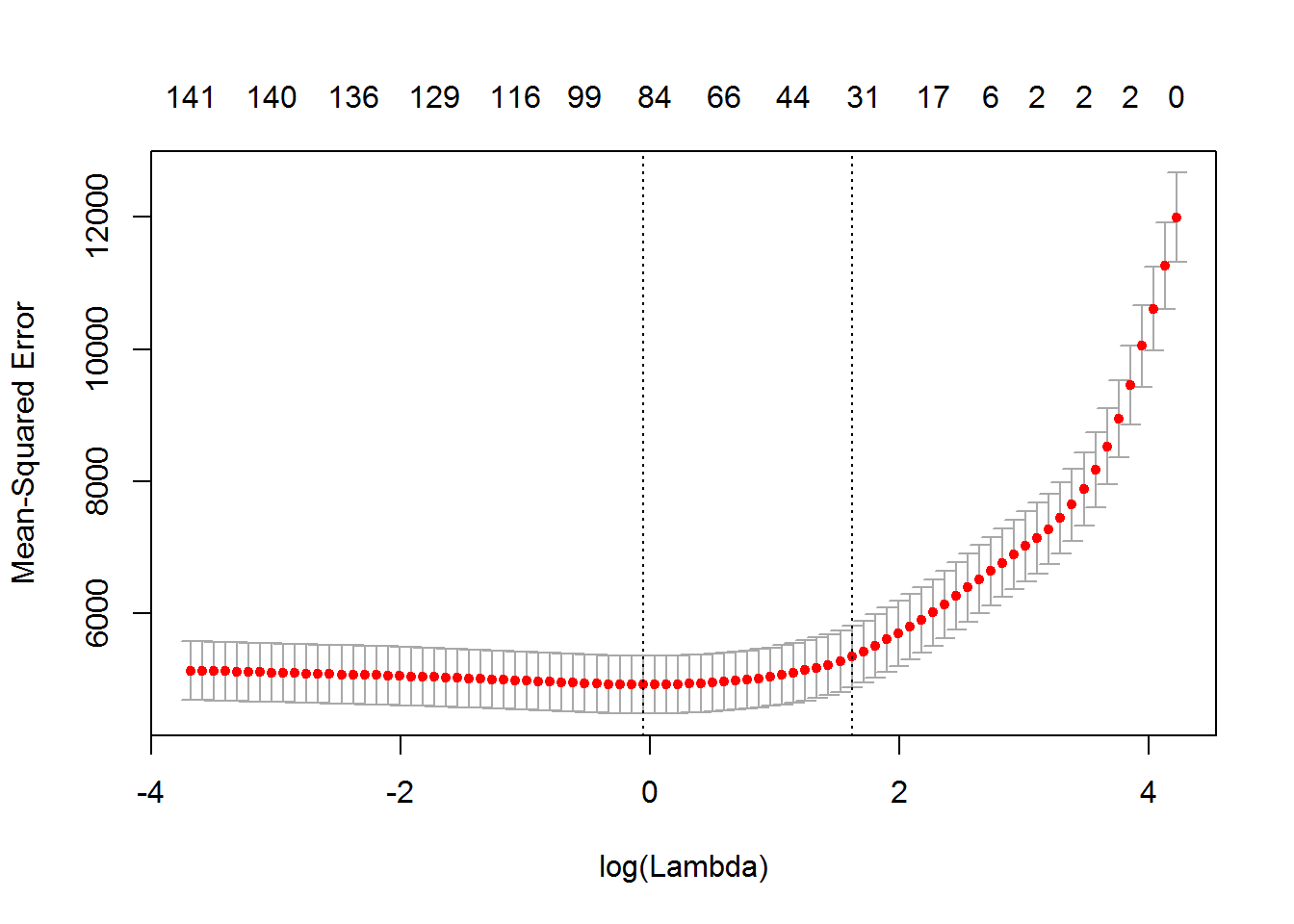The first vertical dotted line shows lambda.min, and the second is lambda.1se. The figure illustrates that we cross-validate to find the “sweet spot” where there’s not too much bias (high lambda) and not too much noise (low lambda). The left-hand side of this graph is flatter than we’d sometimes see, meaning that the unpenalized model may not be too bad. However, increasing lambda increases interpretability at close to no loss in prediction accuracy! Let’s again compare training and test error. Since the predict() function for glmnet objects uses matrices, we can’t use the rmse function like we did before. x_all = model.matrix(~ .-price + accommodates*room_type + property_type*neighbourhood_cleansed + review_scores_rating*neighbourhood_cleansed + accommodates*review_scores_rating,data=listings_big) # Matrix form for combined test and training data listings_big %>% mutate(is_test = 1:nrow(listings_big) %in% listings_big_lm$test$idx, pred = as.vector(predict.cv.glmnet(lasso_price_cv,newx=x_all))) %>% group_by(is_test) %>% summarize(rmse = sqrt(1/length(price)*sum((price-pred)^2))) ## # A tibble: 2 × 2 ## is_test rmse ## <lgl> <dbl> ## 1 FALSE 70.45516 ## 2 TRUE 78.62953 The overfitting problem has gotten better, but hasn’t yet gone away completely. I added a bunch variables for dramatic effect that we could probably screen out before running the LASSO if we really wanted a good model. One more note on cross-validation: the glmnet package has built-in functionality for cross-validation. In situations where that’s not the case, modelr::crossv_kfold() will prepare data for cross validation in a nice way. ## Classification So far we’ve looked at models which predict a continuous response variable. There are many related models which predict categorical outcomes, such as whether an email is spam or not, or which digit a handwritten number is. We’ll take a brief look at two of these: logistic regression and classification trees. ### Logistic Regression Logistic regression is part of the class of generalized linear models (GLMs), which build directly on top of linear regression. These models take the linear fit and map it through a non-linear function. For logistic regression this function is the logistic function, $$f(x) = \exp(x)/(1+\exp(x))$$, which looks like this: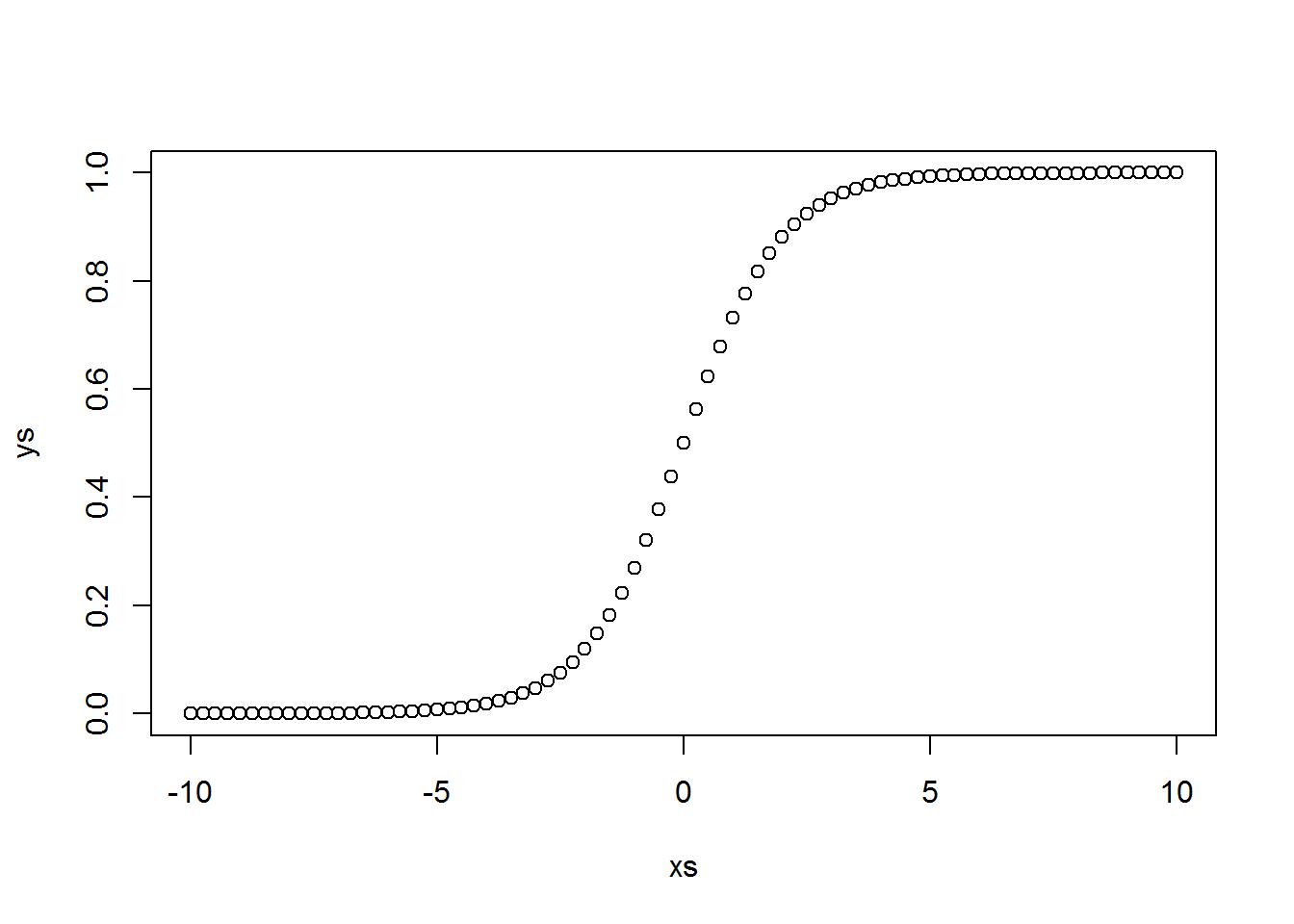Since the function stays between zero and one, it can be interpreted as a mapping from predictor values to a probability of being in one of two classes. Let’s apply this model to the listings data. Let’s try to predict which listings have elevators in the building by price. To make sure we’re asking a sensible question, we’ll only consider apartments. We’ll also filter out price outliers. set.seed(863) listings_glm = listings %>% filter(property_type == "Apartment", price <= 500) %>% resample_partition(c(train=0.7,test=0.3)) Instead of the lm() function, we’ll now use glm(), but the syntax is almost exactly the same: l.glm = glm(amenity_Elevator_in_Building ~ price,family="binomial",data=listings_glm$train)
summary(l.glm)
##
## Call:
## glm(formula = amenity_Elevator_in_Building ~ price, family = "binomial",
##     data = listings_glm$train) ## ## Deviance Residuals: ## Min 1Q Median 3Q Max ## -1.7908 -0.8582 -0.6797 1.1951 1.9612 ## ## Coefficients: ## Estimate Std. Error z value Pr(>|z|) ## (Intercept) -1.987236 0.135344 -14.68 <2e-16 *** ## price 0.006732 0.000641 10.50 <2e-16 *** ## --- ## Signif. codes: 0 '***' 0.001 '**' 0.01 '*' 0.05 '.' 0.1 ' ' 1 ## ## (Dispersion parameter for binomial family taken to be 1) ## ## Null deviance: 1875.1 on 1490 degrees of freedom ## Residual deviance: 1753.9 on 1489 degrees of freedom ## AIC: 1757.9 ## ## Number of Fisher Scoring iterations: 4 Again, we can add predictions to the data frame and plot these along with the actuals, although the result doesn’t look nearly as clean: as.data.frame(listings_glm$train) %>%
mutate(pred = predict(l.glm,data=listings_glm$train,type="response")) %>% ggplot(aes(x=price)) + geom_line(aes(y=pred)) + geom_point(aes(y=amenity_Elevator_in_Building + 0))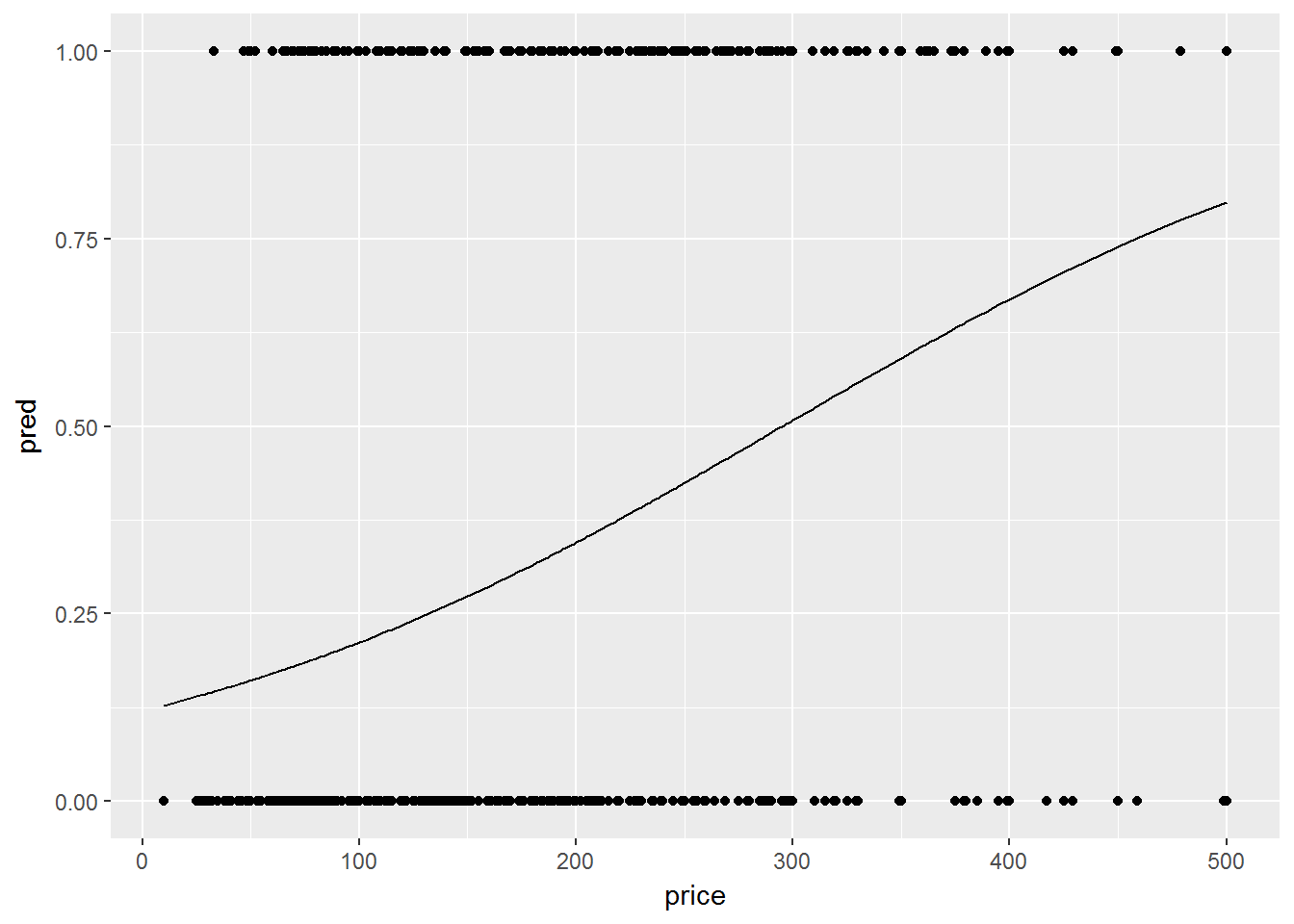One way to get a more informative plot is by using the logi.hist.plot() function in the popbio package. In the meantime, we can explore out-of-sample performance. Ultimately, we want to predict whether or not a listing has an elevator. However, logistic regression gives us something a bit different: a probability that each listing has an elevator. This gives us flexibility in the way we predict. The most natural thing would be to predict that any listing with predicted probability above 0.5 has an elevator, and any listing with predicted probability below 0.5 does not have an elevator. But what if I use a wheelchair and I want to be really confident that there’s going to be an elevator? I may want to use a cutoff value of 0.9 rather than 0.5. In fact, we could choose any cutoff value and have a corresponding prediction model. There’s a really nice metric that measures the quality of all cutoffs simultaneously: AUC, for “Area Under the receiver operating characteristic Curve.” That’s a mouthful, but the idea is simpler: For every cutoff, we’ll plot the false positive rate against the true positive rate and then take the area under this curve. (A positive in our case is a listing that has an elevator. So a true positive is a listing that we predict has an elevator and really does have an elevator, while a false positive is a listing that we predict has an elevator and does not actually have an elevator.) As the cutoff shrinks down from 1 to 0, the rate of total positives will increase. If the rate of true positives increases faster than the rate of false positives, this is one indication that the model is good. This is what AUC measures. The ROCR package is one implementation that allows us to plot ROC curves and calculate AuC. Here’s an example (make sure to install the packages first using install.packages("ROCR")): library(ROCR) preds = predict(l.glm,as.data.frame(listings_glm$test),type="response")
test_elevators = as.data.frame(listings_glm$test)$amenity_Elevator_in_Building
pred_obj = prediction(preds,test_elevators) # Creating a prediction object for ROCR
perf = performance(pred_obj,'tpr','fpr')
plot(perf) # ROC curve